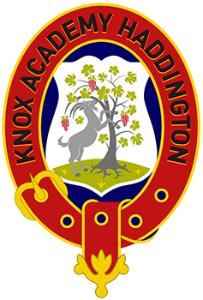# Design

## Learning Intentions

• Describe and compare different means of software design

## Success Criteria

• Be able to describe and use structure diagrams, flowcharts and pseudocode.

## Design notations

• There are 3 different design notations that we need to know at National 5. These are:
• Structure diagrams
• Flowcharts
• Pseudocode

## Design notations: Structure diagrams

• A structure diagram uses a variety of standard symbols with text to represent the order of events required to solve a problem.

## Design notations: Structure diagrams

``````total = 0

for counter in range (0, 10):
number = int(input())

while number < 0 or number > 10:
print("Try again")
number = int(input())

total = total + number

print(total)
``````

## Design notations: Structure diagrams

``````for counter in range (1, 6):
print(counter)
``````

## Design notations: flowcharts

• A flowchart uses a variety of standard symbols with text to represent the order of events required to solve a problem.
• Flowcharts aren't just used in software design

## Design notations: flowcharts

• Special symbols represent each different action or control sequence in the code.

• Create a flow chart or structure diagram for making a cup of tea.
• Create a flow chart or structure diagram that loops, asking the user for a number each time and adding it to a total variable, until the user types in 0. Extension: try creating this in Python.
• Create a flow chart or structure diagram for taking in 10 grades and verifying they are between 0 and 100 (inclusive) and adding them to an array.

## Pseudocode

• Pseudocode is a kind of structured English for describing algorithms and intended for human reading.
• It is a textual design notation.
• It can look like code but it doesn’t have the same strict syntax.
• We should start by thinking about the main steps to solve a problem.  We should then refine the steps so we have one line of pseudocode for each line of program code.

## Pseudocode example

• Lets consider calculating the volume of water in a swimming pool.
What would be the main steps for the problem?

Main Steps
Ask user to enter dimensions of pool in metres
Calculate volume of pool
Display message stating the volume of the pool

## Pseudocode example

• How would we refine these main steps?

Main Steps
1. Ask user to enter dimensions of pool in metres
2. Calculate volume of pool
3. Display message stating the volume of the pool

Refinement
1.1 Ask the user to enter the length of pool
1.2 Ask the user to enter the width of pool
1.3 Ask the user to enter the depth of pool

2.1 Volume = length x width x depth

3.1 Display "The volume of the pool is", volume

## Wireframes

• Wireframes are not just used in web!
• A wireframe is a diagram or sketch of the input and output screens with which the user will interact.
• The wireframe should contain placeholders where data is to be input and labels next to where the output will be.
• It should also include relevant buttons to submit the data.

## Past Paper Questions

Pseudocode was used to design the program. State another technique that could be used to design the program.

1 mark

2019 Q9(a)(i)

## Past Paper Questions

Sam is creating a program to calculate and display the total cost of laying new flooring. Flooring is charged at £15 per square meter and skirting boards are charged at £60 per room.

The total cost is calculated by multiplying the total floor area by 15, then adding the number of rooms requiring skirting multiplied by 60.

Using the information above, design a user interface for the program.

3 marks

2022 10 a)

## Past Paper Questions

Line 3 RECEIVE score FROM KEYBOARD
Line 4 IF score = 5 OR score = 10 OR score = 15 THEN
Line 5   IF NOT(score >= 3 AND score <= 12) THEN
Line 6     SEND "Success" TO DISPLAY
Line 7   END IF
Line 8 END IF

State the score that should be input in Line 3 to display ‘Success’.

1 mark

2022 Q6

Presentation Overview
Design
14:47 | 07-12-2023
!
Keywords
Random selector
Sections
Binary conversion
Denary to binary conversion
Binary to denary conversion
Feedback 👍
Accessibility

Apply a filter:

×
All slideshow files